• +91 9971497814
• info@interviewmaterial.com

# Chapter 8 Binomial Theorem Ex-8.2 Interview Questions Answers

### Related Subjects

Question 1 : Find the coefficient of x5 in (x + 3)8

It is known that (+1)th term, (Tr+1), in the binomialexpansion of (b)n is givenby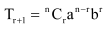.

Assuming that x5 occursin the (r + 1)th term of the expansion (x +3)8, we obtain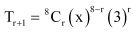Comparing the indices of x in x5 andin Tr +1, we obtain

r = 3

Thus, the coefficient of x5 is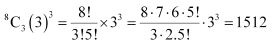Question 2 :

Find the coefficient of a5b7 in(a – 2b)12

It is known that (+1)th term, (Tr+1), in the binomialexpansion of (b)n is givenby.

Assuming that a5b7 occursin the (r + 1)th term of the expansion (a –2b)12, we obtain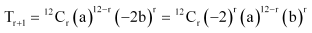Comparing the indices of a and b in a5 bandin Tr +1, we obtain

r = 7

Thus, the coefficient of a5b7 is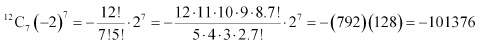Question 3 :

Write the general term in theexpansion of (x2 – y)6

It is known that the generalterm Tr+1 {which is the (+1)th term} in the binomial expansion of (b)n isgiven by.

Thus, the general term in theexpansion of (x2 – y6) is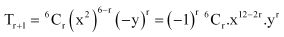Question 4 :

Write the general term in theexpansion of (x2 – yx)12x ≠ 0

It is known that the generalterm Tr+1 {which is the (+1)th term} in the binomial expansion of (b)n isgiven by.

Thus, the general term in theexpansion of(x2 – yx)12 is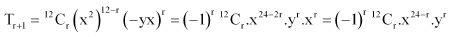Question 5 :

Find the 4th termin the expansion of (x – 2y)12 .

It is known that (+1)th term, (Tr+1), in the binomialexpansion of (b)n is givenby.

Thus, the 4th termin the expansion of (x – 2y)12 is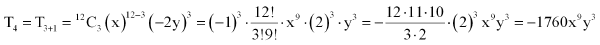Question 6 : Find the 13th term in the expansion of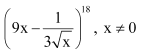.

It is known that (+1)th term, (Tr+1), in the binomialexpansion of (b)n is givenby.

Thus, 13th termin the expansion of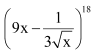is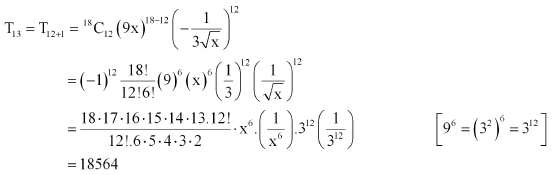Question 7 : Find the middle terms in the expansions of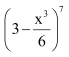It is known that in the expansion of (a + b)n, if n is odd, then there are two middle terms, namely,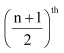term and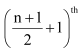term.
Therefore, the middle terms in the expansion ofare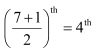term and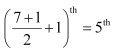term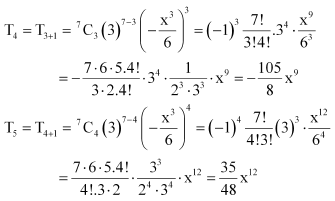Thus, the middle terms in the expansion ofare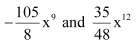.

Question 8 : Find the middle terms in the expansions of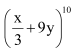It is known that in the expansion (a + b)n, if n is even, then the middle term is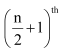term.
Therefore, the middle term in the expansion ofis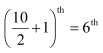term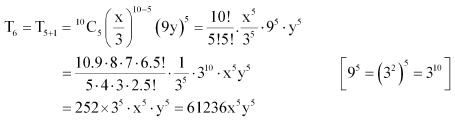Thus, the middle term in theexpansion ofis 61236 x5y5.

Question 9 :

In the expansion of (1 + a)m+ n, prove that coefficients of am and an areequal.

It is known that (+1)th term, (Tr+1), in the binomialexpansion of (b)n is givenby.

Assuming that am occursin the (r + 1)th term of the expansion (1 + a)m + n,we obtain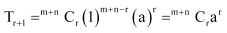Comparing the indices of a in am andin T+ 1, we obtain

r = m

Therefore, the coefficientof am is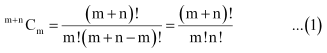Assuming that an occursin the (k + 1)th term of the expansion (1 + a)m+n,we obtain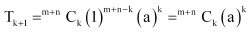Comparing the indices of a in an andin Tk + 1, we obtain

k = n

Therefore, the coefficientof an is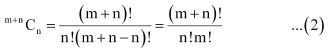Thus, from (1) and (2), it can beobserved that the coefficients of am and an inthe expansion of (1 + a)m + n areequal.

Question 10 :

The coefficients of the (r –1)thrth and (r + 1)th termsin the expansion of

(x + 1)n arein the ratio 1:3:5. Find n and r.

It is known that (+1)th term, (Tk+1), in the binomialexpansion of (b)n is givenby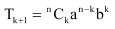.

Therefore, (r – 1)th termin the expansion of (x + 1)n is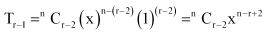r th termin the expansion of (x + 1)n is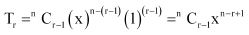(r + 1)th termin the expansion of (x + 1)n is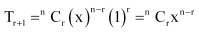Therefore, the coefficients ofthe (r – 1)thrth, and (r +1)th terms in the expansion of (x + 1)n are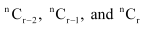respectively. Since thesecoefficients are in the ratio 1:3:5, we obtain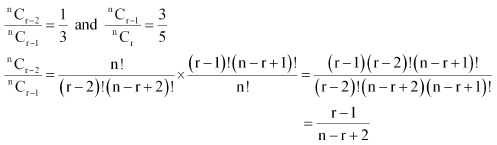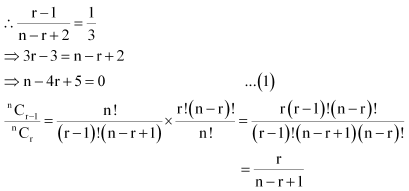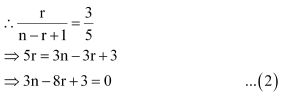Multiplying (1) by 3 andsubtracting it from (2), we obtain

4– 12 = 0

r = 3

Putting the value of r in(1), we obtain

n –12 + 5 = 0

n = 7

Thus, = 7and r = 3

Todays Deals### Chapter 8 Binomial Theorem Ex-8.2 Contributorskrishan

Name:
Email:

# Latest News# 9000 interview questions in different categories